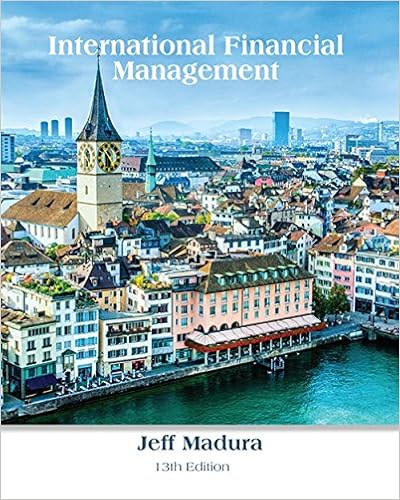# Four a consider the following relationships developed

• Test Prep
• MandyZhu
• 6

This preview shows page 5 - 6 out of 6 pages.

##### We have textbook solutions for you!
The document you are viewing contains questions related to this textbook.The document you are viewing contains questions related to this textbook.
Chapter 18 / Exercise 17
International Financial ManagementExpert Verified
QUESTION FOUR (a) Consider the following relationships developed in the ECON 202 Course: t - t-1 = - (ut - uut - ut-1 = - (gyt- where t is a time subscript denoting a year, =actual inflation rate, u= actual unemployment rate, un= natural unemployment rate, ytg= actual real gdp growth rate, yg= normal real gdp growth rate. Assume that in the current year t = t-1= 3%, ut= un= 6%, and gyt = Suppose that a government wishes to reduce inflation from 3% in the current year to 2% in year t+1. Suppose the government’s policy advisors assume that = 0.5 and  They accordingly recommend that the growth rate of real gdp needs to be reduced by 2% to 1% growth in year t+1 in order to reach the inflation target of 2 % in year t+1. Assume the government follows this advice. (i) Compute and explain what happens to the actual unemployment rate in year t+1 if the Okun Law coefficient, , were to be 0.25 rather than 0.5.(3 MARKS) (ii) If the Okun Law coefficient were in fact 0.5, compute and explain what happens to inflation in year t+1, relative to the announced inflation target, if the value of the Phillips Curve (PC) coefficient, , were to be 0.5 instead of 1.0. (3 MARKS) (iii) Compute and explain what happens to the range of inflation rates, as the range of possible values of and both vary simultaneously over the two values given in (i) for and in (ii) for .(3 MARKS) (iv) Comment briefly on the potential implications of the results in (iii) for policy credibility, in the context of New Zealand’s December 2008 Policy Targets Agreement (PTA) under the Reserve Bank of New Zealand Act, 1989. (3 MARKS) n)yg) yg= 3%. QUESTION FOUR CONTINUED ON NEXT PAGE
##### We have textbook solutions for you!
The document you are viewing contains questions related to this textbook.The document you are viewing contains questions related to this textbook.
Chapter 18 / Exercise 17
International Financial ManagementExpert Verified
•••